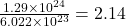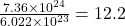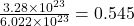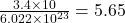## 1. How many moles are in the following: a. 1.29 x 1024 hydrogen atoms in H b. 7.36 x 1024 oxygen atoms c. 3.2

Question

1. How many moles are in the following:
a.
1.29 x 1024 hydrogen atoms in H
b. 7.36 x 1024 oxygen atoms
c. 3.28 x 1023 Na atoms
2. How many molecules are in 23.0 moles of oxygen?
3. How many moles are in 3.4 x 10molecules of H,SO,?
4. How many atoms are in 3.0 mole of Na?
5. How many molecules are in 200 moles of 03?

in progress 0
6 months 2021-07-19T00:50:20+00:00 1 Answers 130 views -1

## Answers ( )

Part (1a): The number of moles are 2.14 moles.

Part (1b): The number of moles are 12.2 moles.

Part (1c): The number of moles are 0.545 moles.

Part 2: The number of molecules are 1.38 × 10²⁵.

Part 3: The number of moles are 5.65 moles.

Part 4: The number of atoms are 1.81 × 10²⁴.

Part 5: The number of molecules are 1.20 × 10²⁶.

Explanation:

According to the mole concept:

1 mole of substance contains 6.022 × 10²³ number of atoms or molecules.

Part (1a): 1.29 × 10²⁴ hydrogen atoms in H

As, 6.022 × 10²³ number of hydrogen atoms present in 1 mole

So, 1.29 × 10²⁴ number of hydrogen atoms present inmole

Part (1b): 7.36 × 10²⁴ oxygen atoms

As, 6.022 × 10²³ number of oxygen atoms present in 1 mole

So, 7.36 × 10²⁴ number of oxygen atoms present inmole

Part (1c): 3.28 × 10²³ Na atoms

As, 6.022 × 10²³ number of Na atoms present in 1 mole

So, 3.28 × 10²³ number of Na atoms present inmole

Part 2:

As, 1 mole of oxygen contains 6.022 × 10²³ number of molecules

So, 23.0 moles of oxygen contains 23.0 × 6.022 × 10²³ = 1.38 × 10²⁵ number of molecules

Part 3:

As, 6.022 × 10²³ number of H₂SO₄ molecules present in 1 mole

So, 3.4 × 10 number of H₂SO₄ molecules present inmole

Part 4:

As, 1 mole of Na contains 6.022 × 10²³ number of atoms

So, 3.0 moles of Na contains 3.0 × 6.022 × 10²³ = 1.81 × 10²⁴ number of atoms

Part 5:

As, 1 mole of O₃ contains 6.022 × 10²³ number of molecules

So, 200 moles of O₃ contains 200 × 6.022 × 10²³ = 1.20 × 10²⁶ number of molecules